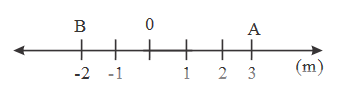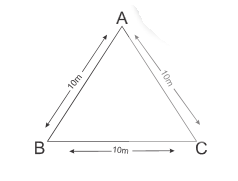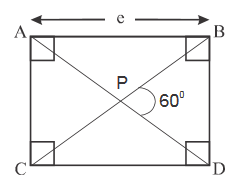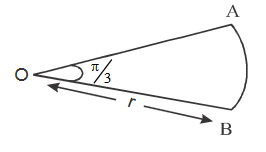1.  A branch of physics dealing with motion without considering its causes is known as

A. Kinematics

B. Dynamics

C. Hydrodynemics

D. Mechanics

2.  Mechanics is a branch of physics. This branch is

A. Kinematics without dynamics

B. Dynamics without Kinematics

C. Kinematics and dynamics

D. Kinematics or dynamics

3.  To locate the position of the particle we need

A. A frame of reference

B. Direction of the particle

C. Size of the particle

D. Mass of the particle

4.  Frame of reference is a _____ and a _____ from where an observer takes his observation,

A. Place, Size

B. Size, Situation

C. Situation, Size

D. Place, Situation

5.As shown in the figure a particle moves from 0 to A, and then A to B. Find path length and displacement.

A. 2m, –2m

B. 8m, –2m

C. 2m, 2m

D. 8m, –8m

6.

A particle moves from A to B and then it moves from B to C as shown in figure. Calculate the ratio between path lenghth and displacement.A. 2

B. 1

C. $$\frac{1}{2}$$

D. $$\infty$$

7.

A particle moves from A to P and then it moves from P to B as shown in the figure. Find path length and dispalcement.A. $$\frac{2e}{\sqrt{3}} ,e$$

B. $$\frac{e}{\sqrt{3}} ,e$$

C. $$2e,e$$

D. $$e,\frac{2e}{\sqrt{3}}$$

8.  A car goes from one end to the other end of a semicircular path of diameter ‘d’. Find the ratio between path length and displacement.

A. $$\frac{3\pi }{\sqrt{2}}$$

B. $$\pi$$

C. $$2$$

D. $$\frac{\pi }{\sqrt{2}}$$

9.  A particle goes from point A to B. Its displacement is X and path length is y. So $$\frac{x}{y}$$

A. $$> 1$$

B. $$< 1$$

C. $$\geqslant 1$$

D. $$\leqslant 1$$

Explanation :

Path length is always greater or equal to displacement

10.

As shown in the figure a partricle statrs its motion from O to A. And then it moves from A to B. $$\overline{AB}$$ is an arc find the Path lengthA. $$2r$$

B. $$r+\frac{\pi }{3}$$

C. $$r\left ( 1+\frac{\pi }{3} \right )$$

D. $$\frac{\pi }{3}(r+1)$$